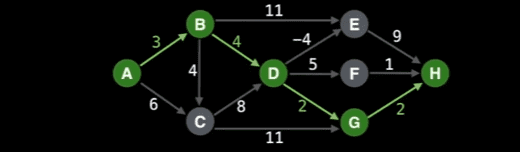# Using Single Source Shortest Path Algorithm to find the longest path

• Comp Sci
• Monsterboy

#### Monsterboy

Homework Statement
Using Single Source Shortest Path Algorithm to find the longest path
Relevant Equations
First multiply the edge weights by -1 and find the shortest path, then multiply the result by -1 again.
This is the weighted, directed acyclic graph I created in JavaScript

Code:
class WeightedDirectedGraph {
constructor() {
}

}
}

this.adjacencyList[node1].push({node: node2, direction: direction, weight: weight });
this.adjacencyList[node2].push({node: node1, direction: direction, weight: weight });
}

getEdgesFromNode(node) {
let edges = []
for (let i = 0; i < this.adjacencyList[node].length; i++) {
}
}
return edges;
}
}

let graph = new WeightedDirectedGraph();

graph.addEdge("A", "B", {from: "A", to: "B"}, 3)
graph.addEdge("A", "C", {from: "A", to: "C"}, 6)
graph.addEdge("B", "C", {from: "B", to: "C"}, 4)
graph.addEdge("B", "D", {from: "B", to: "D"}, 4)
graph.addEdge("B", "E", {from: "B", to: "E"}, 11)
graph.addEdge("C", "D", {from: "C", to: "D"}, 8)
graph.addEdge("C", "G", {from: "C", to: "G"}, 11)
graph.addEdge("D", "E", {from: "D", to: "E"}, -4)
graph.addEdge("D", "F", {from: "D", to: "F"}, 5)
graph.addEdge("D", "G", {from: "D", to: "G"}, 2)
graph.addEdge("E", "H", {from: "E", to: "H"}, 9)
graph.addEdge("F", "H", {from: "F", to: "H"}, 1)
graph.addEdge("G", "H", {from: "G", to: "H"}, 2)

I will attach an image to visualize this graphThis is the topological sort code

Code:
function topSort(graph) {
let V = {};  // visited nodes list
let ordering = [];
for (let node in graph.adjacencyList) {
V[node] = false;
ordering.push(0);
}
let i = N - 1;
for (let node in graph.adjacencyList) {
if(V[node] === false) {
i = dfs(i, node, V, ordering, graph)
}
}
return ordering;
}

function dfs(i, node, V, ordering, graph) {
V[node] = true;
let edges = graph.getEdgesFromNode(node)
for (let k = 0; k < edges.length; k++) {
if (V[edges[k].direction.to] === false) {
i = dfs(i, edges[k].direction.to, V, ordering, graph)
}
}
ordering[i] = node;
return i - 1;
}

function dagShortestPath(graph, startNode) {
let topSortResult = topSort(graph);
let distances = {};
topSortResult.forEach((node) => {
distances[node] = Infinity;
})

distances[startNode] = 0;
for( let i = 0; i < topSortResult.length; i++) {
let nodeIndex = topSortResult[i];
let edges = graph.getEdgesFromNode(nodeIndex);
if (edges.length !== 0) {
let newDist;
for (let i = 0; i < edges.length; i++) {
newDist = distances[nodeIndex] + edges[i].weight;
if (distances[edges[i].direction.to] === Infinity) {
distances[edges[i].direction.to] = newDist;
}
else if (newDist > -1) {
distances[edges[i].direction.to] = Math.min(distances[edges[i].direction.to], newDist);
}
}
}
}
return distances;
}
I tested the code for the shortest path from node A to all other nodes, I am getting it right.
The output is { A: 0, B: 3, C: 6, D: 7, G: 9, F: 12, E: 3, H: 11 }

This is the code for the longest path.

Code:
function LongestPath(graph, startNode) {
let topSortResult = topSort(graph);
let distances = {};
topSortResult.forEach((node) => {
distances[node] = Infinity;
})
distances[startNode] = 0;
// multiply all edge weights by -1
for (let node in graph.adjacencyList) {
for (let i = 0; i < graph.adjacencyList[node].length; i++) {
}
}
let longestDistances = dagShortestPath(graph, startNode);
// multiply all edge weights by -1 after getting longest path
for (let node in graph.adjacencyList) {
for (let i = 0; i < graph.adjacencyList[node].length; i++) {
}
}
for (let node in longestDistances) {
longestDistances[node] *= -1;
}
return longestDistances;
}

I am getting this wrong, even though the only change is the multiplication of the edge weights with -1.
Output is { A: -0, B: 3, C: 6, D: 7, G: 17, F: 12, E: 14, H: 19 } although for some nodes the output is right.

I took the image and the idea of multiplying by -1 from this free YouTube course on graph algorithms from freeCodeCamp.org channel -

### Algorithms Course - Graph Theory Tutorial from a Google Engineer​

Last edited:

If you set the language to javascript as in the code below it is much easier to read.

JavaScript:
class WeightedDirectedGraph {
constructor() {
}

}
}

this.adjacencyList[node1].push({node: node2, direction: direction, weight: weight });
this.adjacencyList[node2].push({node: node1, direction: direction, weight: weight });
}

getEdgesFromNode(node) {
let edges = []
for (let i = 0; i < this.adjacencyList[node].length; i++) {
}
}
return edges;
}
}

let graph = new WeightedDirectedGraph();

graph.addEdge("A", "B", {from: "A", to: "B"}, 3)
graph.addEdge("A", "C", {from: "A", to: "C"}, 6)
graph.addEdge("B", "C", {from: "B", to: "C"}, 4)
graph.addEdge("B", "D", {from: "B", to: "D"}, 4)
graph.addEdge("B", "E", {from: "B", to: "E"}, 11)
graph.addEdge("C", "D", {from: "C", to: "D"}, 8)
graph.addEdge("C", "G", {from: "C", to: "G"}, 11)
graph.addEdge("D", "E", {from: "D", to: "E"}, -4)
graph.addEdge("D", "F", {from: "D", to: "F"}, 5)
graph.addEdge("D", "G", {from: "D", to: "G"}, 2)
graph.addEdge("E", "H", {from: "E", to: "H"}, 9)
graph.addEdge("F", "H", {from: "F", to: "H"}, 1)
graph.addEdge("G", "H", {from: "G", to: "H"}, 2)

And if you add indentation it is easier still:
JavaScript:
class WeightedDirectedGraph {
constructor() {
}

}
}

this.adjacencyList[node1].push({ node: node2, direction: direction, weight: weight });
this.adjacencyList[node2].push({ node: node1, direction: direction, weight: weight });
}

getEdgesFromNode(node) {
let edges = []
for (let i = 0; i < this.adjacencyList[node].length; i++) {
}
}
return edges;
}
}

This bug might be hard to track down. I suggest you focus on what each line of code is doing when it decides that the longest path from A to C is 6 instead of 7 as this is the simplest "error".

•Monsterboy
Is the idea of multiplying the edge weights by -1 to find the longest path right ? Does it actually work ?

In the graph class definition, this method was returning wrong edges.
JavaScript:
 getEdgesFromNode(node) {
let edges = []
for (let i = 0; i < this.adjacencyList[node].length; i++) {
if(this.adjacencyList[node][i].direction.from === node) {   // this condition fixed the issue.
}
•